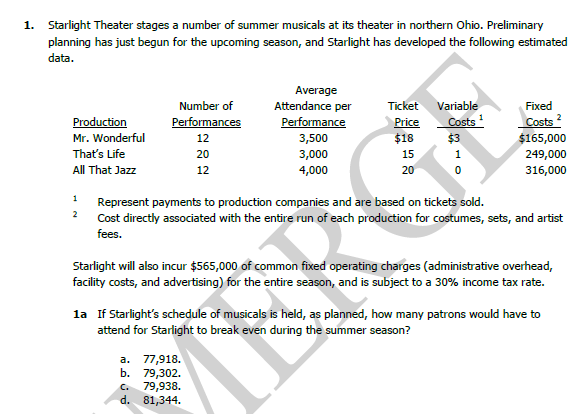# CMA Study Group

View Only

## Part2 Section C#### Rajesh Alexander21 days ago#### Ashvin Kulkarni21 days ago#### Rajesh Alexander21 days ago#### Ashvin Kulkarni21 days ago• #### 1.  Part2 Section C

Posted 21 days ago• #### 2.  RE: Part2 Section C

Posted 21 days ago
Can you confirm is the answer is C. 79,938?

If yes I will try to explain as per my understanding

------------------------------
Ashvin Kulkarni
Accountant
Pune
India
------------------------------

• #### 3.  RE: Part2 Section C

Posted 21 days ago
Hi Ashwin,
Unfortunately I don't have the answer.

Ca you explain your understanding with option C

• #### 4.  RE: Part2 Section C

Posted 21 days ago
So the first thing we need to do is to arrive at the wighted average of contribution margin.
To do so, we will have to allocate weights to the contribution margin per unit of all the three productions.

Weights should be assigned on the basis on tickets sold for each performance as it is mentioned that variable costs are based on the tickets sold.

So to do so we have to multiply the 'Average attendance per performance' by the 'number of performances'.

For example
1) Mr Wonderful it will be 12 * 3,500 = 42,000      (Contribution margin per unit : 18 - 3 = 15 i.e., Price - Variable cost)
2) Thats Life  20 * 3,000 = 60,000                         (Contribution margin per unit : 15 - 1 = 14 i.e., Price - Variable cost)
3) All That Jazz 12 * 4,000 = 48,000                      (Contribution margin per unit : 20 - 0 = 20 i.e., Price - Variable cost)

So now we have weights as 42+60+48 = 150

Further we have to multiply contribution margin per unit by its weight for each production
as,
Mr. Wonderful :  (15*42)/150 = 4.2
Thats Life :         (14*60)/150 = 5.6
All That Jazz :    (20*48)/150 = 6.4

Total weighted average contribution margin = \$16.2

Now as we have to get to the Break Even for attendees:
we have to simply apply the formula of breakeven : Fixed Cost / Contribution Margin/Unit (here, it will be weighted average contribution margin/unit)

Fixed costs are \$165,000 (Mr, Wonderful) + \$249,000 (Thats Life) + \$316,000 (All That Jazz) + \$565,000 (Common fixed operating costs) = \$1,295,000

Putting in formula of Breakeven it will be \$1,295,000 (Fixed costs) / \$16.2 (Weighted avg. contribution margin per unit) = 79,938

*Note - this is as per my knowledge. It would be pleasure and helpful if anyone confirms this answer and if wrong please correct.

Thanks

------------------------------
Ashvin Kulkarni
Accountant
Pune
India
------------------------------

• #### 5.  RE: Part2 Section C

Posted 20 days ago
Thanks Ashvin well explained